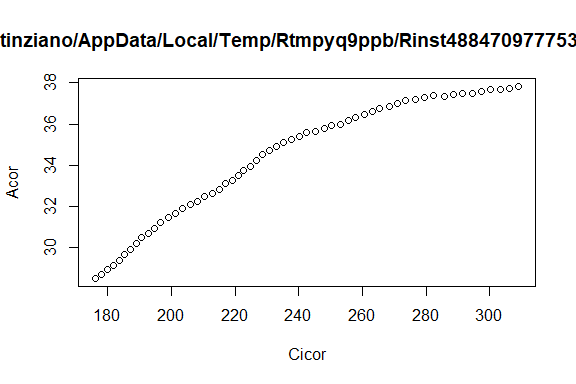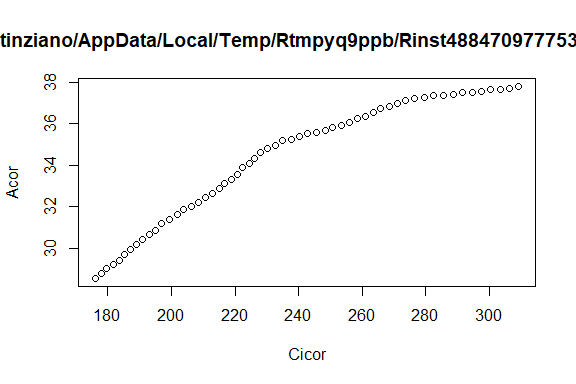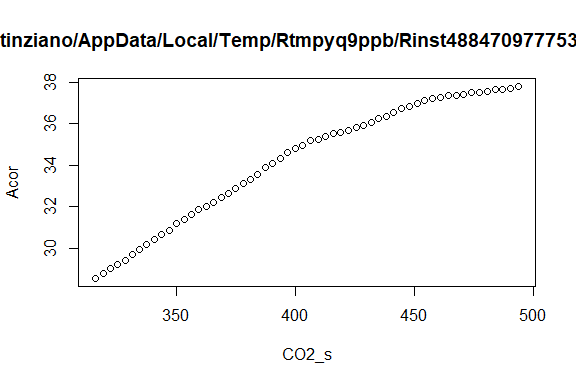racir - Rapid A/Ci response analysis

2020-07-29

This vignette explains how to process rapid A/Ci response (RACiR) data with the ‘racir’ package in R. It is written to be usable for beginners in R.

After installing the package from CRAN with ‘install.packages(“racir”)’, load the package :

library(racir)

##Reading in Data In the examples below I will use three built-in data files in the ‘racir’ package: ‘cal’, ‘poplar_1’, and ‘poplar_2’. These are LI6800 data files in a tab-delimited format. These files can be called in using the code:

system.file(“extdata”, “FILENAME”, package = “racir”)

where FILENAME is one of the named files above.

The following example is to read in a data file from the LI6800. Note that this is not restricted to RACiR data, but can be used for reading LI6800 files in general.

data <- read_6800(system.file("extdata", "cal", package = "racir"))

##Checking Calibration Data Since RACiR precludes matching the sample and reference gas analyzers, an empty chamber RACiR needs to be run and used to correct the leaf sample data. First however, it is necessary to ensure that only usable calibration data are retained. The following code outputs three graphs: ‘Check cutoffs’ helps you to visually determine where the unusable shoulder portions are of the RACiR. ‘Confirm cutoffs’ helps you to verify that your cutoff arguments are appropriate. ‘Calibration Fits’ shows you how each polynomial fits the data.

data <- read_6800(system.file("extdata", "cal", package = "racir"))
#Run calibration check
racircalcheck(data = data)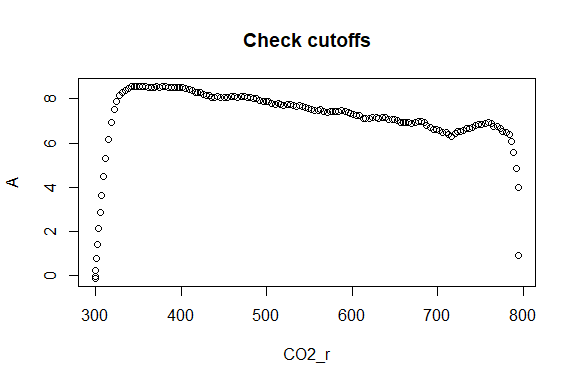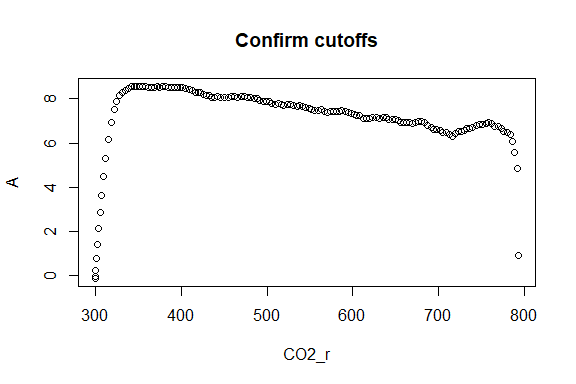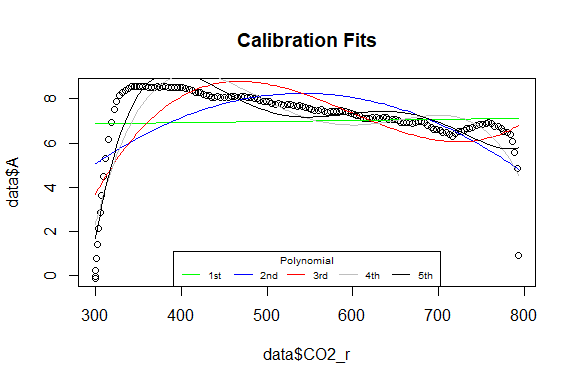##  cal5th

In the above example, there are shoulders in the A versus CO2_r response. These shoulders represent less predictable chamber mixing at the beginning and end of RACiRs. We can cut them out using the ‘mincut’ and ‘maxcut’ arguments.

data <- read_6800(system.file("extdata", "cal", package = "racir"))
#Run calibration check
racircalcheck(data = data,
mincut = 350,
maxcut = 780)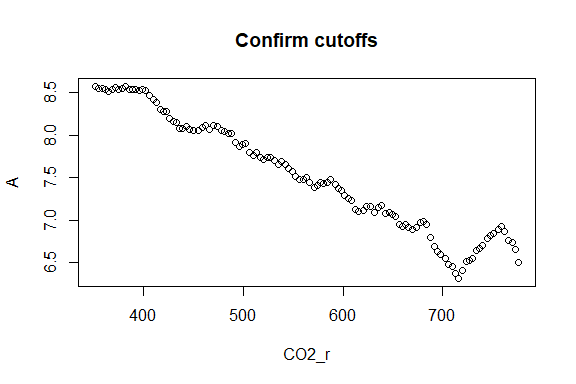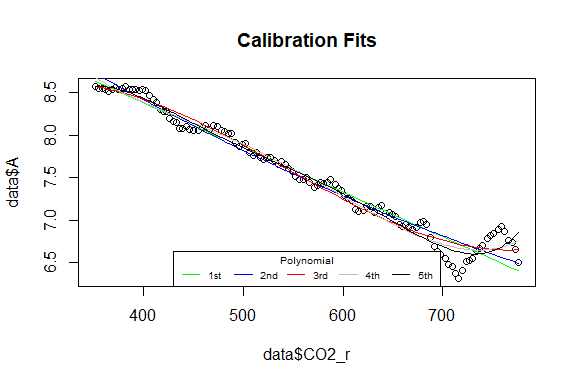##  cal5th
#If using the interval option, use the following function. See below
#for more detailed description
mincut = 350,
maxcut = 780)
##  cal4th
##  cal5th
##  cal5th
##  cal5th
##  cal5th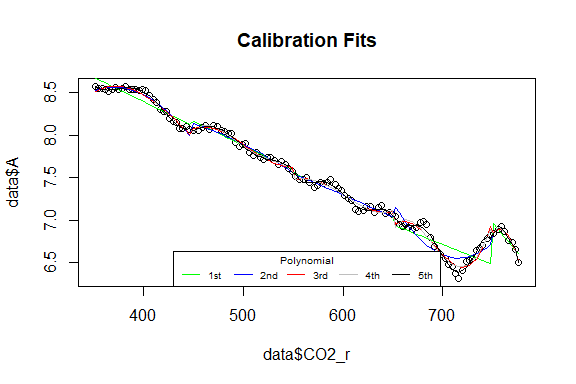From the ‘Check cutoffs’ and ‘Calibration Fits’ graphs, we can see that the cutoffs for CO2_r of 350 and 780 narrow the calibration file to a predictable response.

##Correcting RACiR Files The following code can be used to correct a single RACiR data file based on a paired calibration file. This code should be used after running ‘racircalcheck’ to determine appropriate cutoffs. The ‘racircal’ function is most appropriate when you have one calibration file per leaf RACiR.

data <- read_6800(system.file("extdata", "poplar_2", package = "racir"))
caldata <- read_6800(system.file("extdata", "cal", package = "racir"))

data_corrected <- racircal(data = data, caldata = caldata,
mincut = 350, maxcut = 780)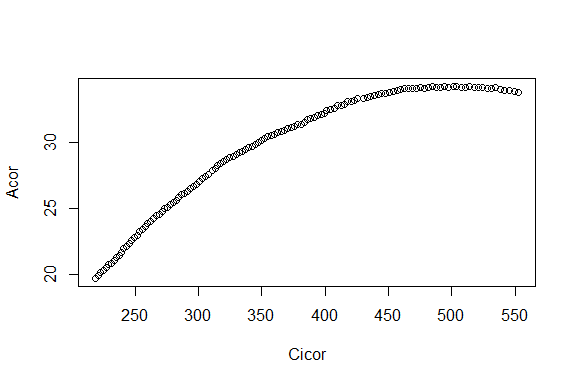Now in some cases, an interval correction may be more appropriate, depending on how the two infrared gas analyzers are calibrated relative to one another. The following “advanced” approach splits the reference CO2 into intervals, then fits a calibration curve for each interval to correct the data. The default interval is 100 ppm

data <- read_6800(system.file("extdata", "poplar_2", package = "racir"))
caldata <- read_6800(system.file("extdata", "cal", package = "racir"))

data_corrected <- racircal_advanced(data = data, caldata = caldata,
mincut = 350, maxcut = 780,
digits = -2)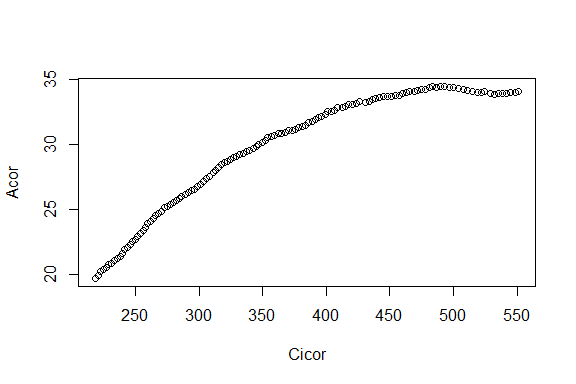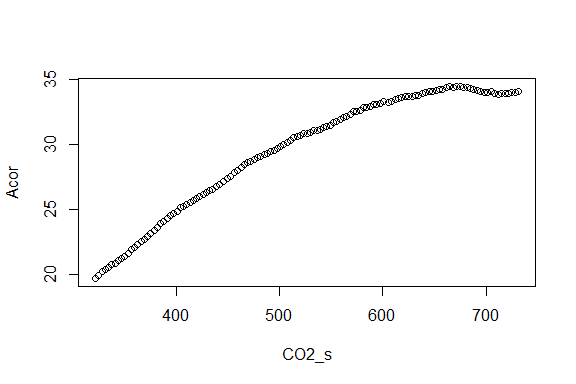If there is more than one leaf RACiR per calibration file, then a batch analysis can be performed using the ‘racircalbatch’ function.

#Create a list of files
files <- c(system.file("extdata", "poplar_1", package = "racir"),
system.file("extdata", "poplar_2", package = "racir"))
data <- vector("list", length(files))
for(i in seq_along(files)){
names(data)[i] <- files[i]
}

caldata <- read_6800(system.file("extdata", "cal", package = "racir"))

#Batch calibration with normal algorithm
output <- racircalbatch(caldata = caldata, data = data,
mincut = 350, maxcut = 780, title = files)